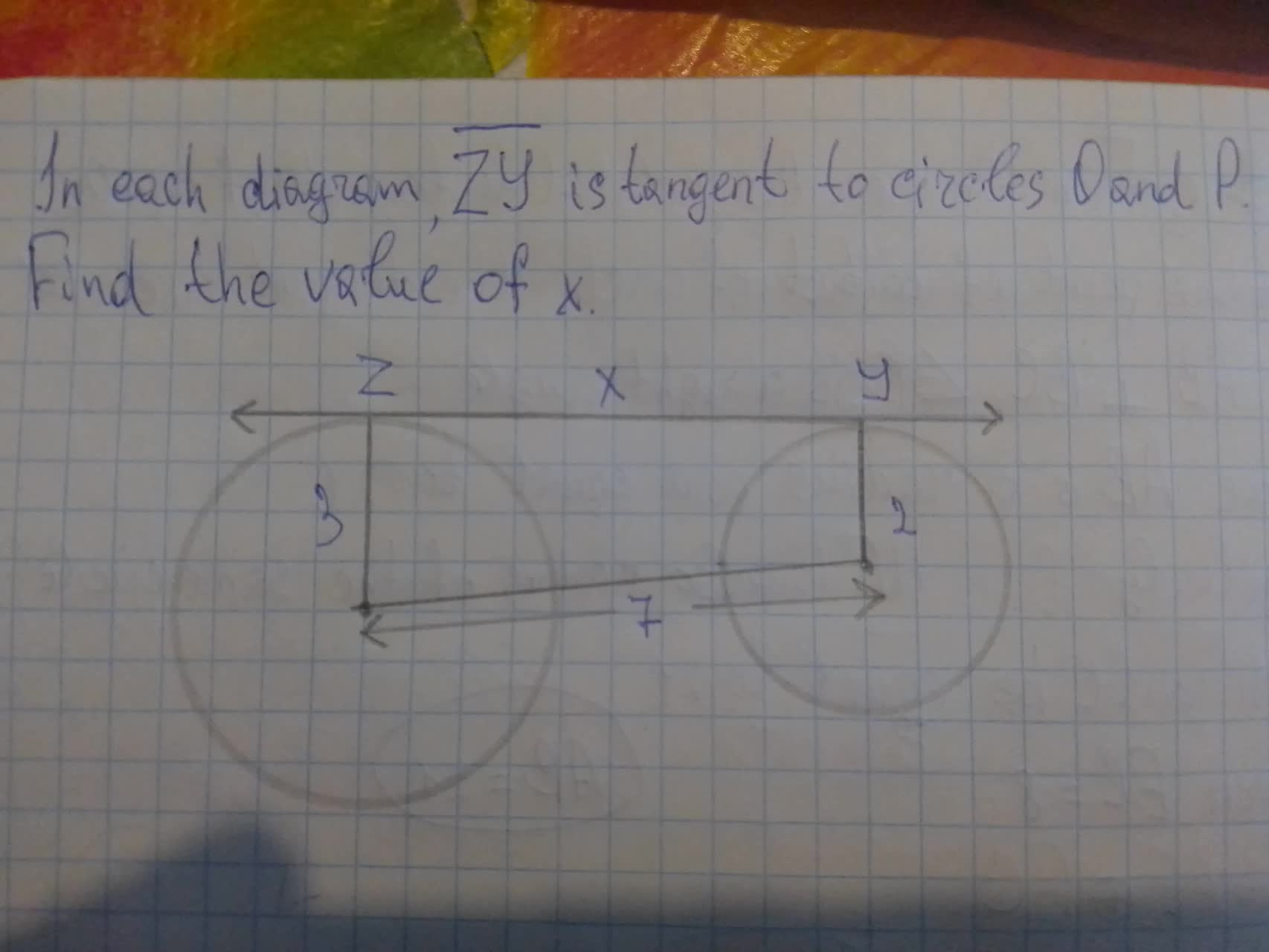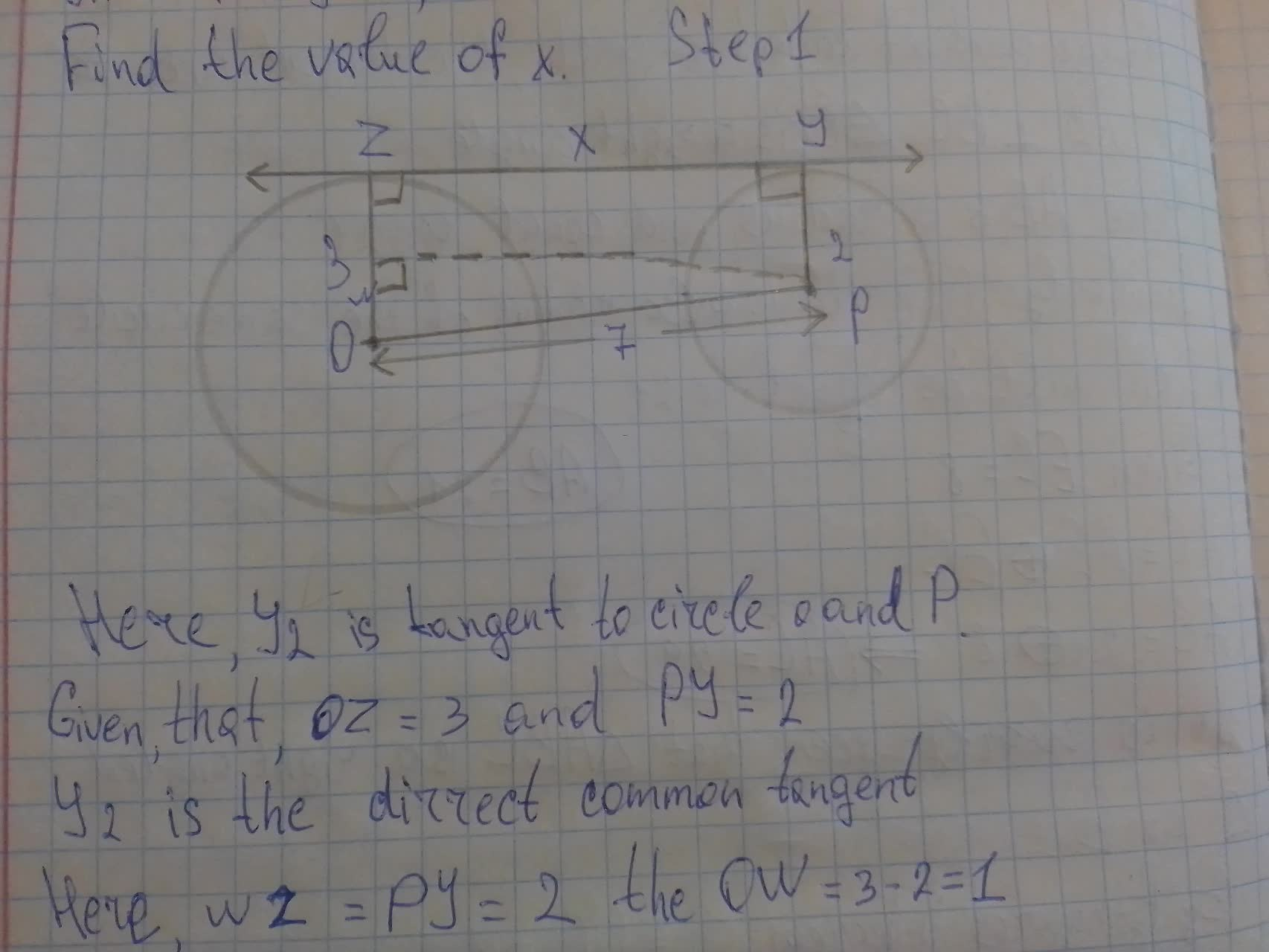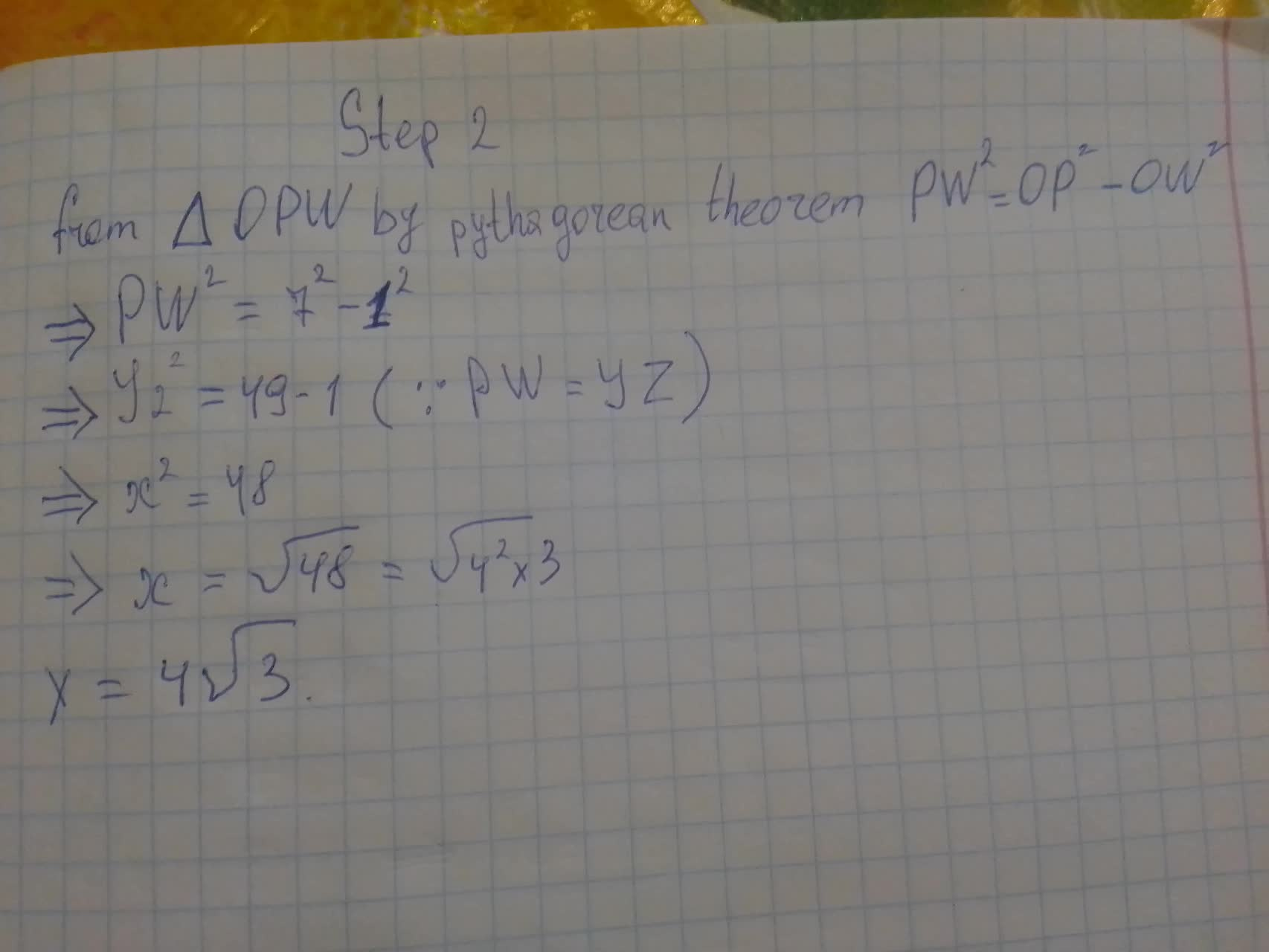In each diagram, \overline{ZY} is tangent to circles O and P. Find the value of x.Tyra 2021-08-12 Answered
In each diagram, $$\displaystyle\overline{{{Z}{Y}}}$$ is tangent to circles O and P. Find the value of x.• Questions are typically answered in as fast as 30 minutes

Solve your problem for the price of one coffee

• Math expert for every subject
• Pay only if we can solve itBentley Leach

Calculation: